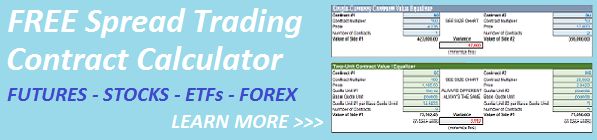Select Page< Back to Case 2

### How to Equalize Spread Legs When the Securities are Denominated in Different Currencies

This is the base case where both securities are denominated in different currencies and a currency transformation is required in addition to a Contract Multiplier conversion..  You cannot equalize the two contracts without including a Currency transformation to a Base Currency.

Suppose you are spread trading two equity index futures contracts, the JPY-denominated TOPIX 150 future listed on the OSE (ticker JTPX) and the USD-denominated E-Mini S&P 500 future listed on the CME (ticker ES).  Let’s also say you want to normalize both contracts to U.S. Dollars (the Base Currency Unit).

For our example, let’s use these values:

• Currency = JPY (JTPX) and USD (ES)
• Currency Value vs. Base Currency (your trading currency) = 119.74 JPY per USD   SEE http://www.oanda.com/currency/converter/
• Quote Unit = index points for both contracts
• Minimum Tick Value = 1 index point (JTPX) and 0.25 index point (ES)
• Contract Multiplier = 10,000 (JTPX) and 50 (ES)
• Price = 1403.00 (JTPX) and 2058.00 (ES)

Here’s the contract calculation to normalize both legs.  Simply adjust the number of contracts on each leg to minimize the absolute variance in net exposures.

JTPX leg exposure = (10,000 x 1403.00 x contracts)/119.74

ES leg exposure = 50 x 2,058 x contracts